##### Physics I Workbook For Dummies with Online Practice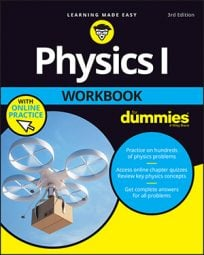When you have objects in motion, you have kinetic energy. When, for example, you slide an object on a frictionless surface, the work you do goes into the object’s kinetic energy:

W = KEfKEi

If you have an object with mass m moving at speed v, its kinetic energy is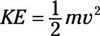That’s the energy of motion.

## Sample question

1. Say that you push a spaceship, mass 1,000.0 kg, by applying a force of 1.00 x 104 N for 1.00 m. How fast does the spaceship end up traveling?

The correct answer is 4.47 m/s.

1. Find the total work done:

(1.00 x 104 N)(1.00 m) = 1.00 x 104 J
2. The work done on the spaceship goes into its kinetic energy, and its initial kinetic energy is 0, so its final kinetic energy is 1.0 x 104 J.

3. Use the equation for kinetic energy and solve for v: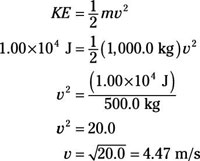The final speed is 20.01/2 = 4.47 m/s.

## Practice questions

1. You’re applying 600.0 N of force to a car with a mass of 1,000.0 kg. You’re traveling a distance of 100.0 m on a frictionless, icy road. What is the car’s final speed?

2. You’re ice skating and traveling at 30.0 m/s. If your mass is 65 kg, what is your kinetic energy?

3. You’re traveling in a car at 88 m/s. If you have a mass of 80.0 kg and the car has a mass of 1,200.0 kg, what is the total kinetic energy of you and the car combined?

4. You’re applying 3,000.0 N of force to a hockey puck with a mass of 0.10 kg. It travels over a distance of 0.10 m on a frictionless ice rink. What is the puck’s final speed?

Following are answers to the practice questions:

1. 11 m/s

1. Find the total work done:

(600 N)(100 m) = 6.0 x 104 J

2. The work done on the car goes into its kinetic energy, so its final kinetic energy is 6.0 x 104 J.

3. Use the equation for kinetic energy and solve for v: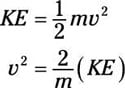4. Plug in the numbers: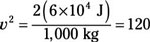The final speed = 1201/2 = 11 m/s.

2. 2.9 x 104 J

1. The equation for kinetic energy is2. Plug in the numbers: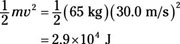3. 4.9 x 106 J

1. The equation for kinetic energy is2. Plug in the numbers: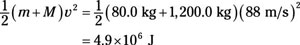4. 77 m/s

1. Find the total work done:

(3,000 N) (0.10 m) = 300 J

2. The work done on the puck goes into its kinetic energy, so its final kinetic energy is 300 J.

3. Use the equation for kinetic energy and solve for v: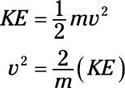4. Plug in the numbers: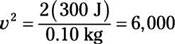The final speed = 6,0001/2 = 77 m/s.